Lift a chestnut! Tableau Tips (126): Learn a few commonly used date functions

When our data source has a date field, it is necessary to use the function to calculate the date according to the analysis required by the date.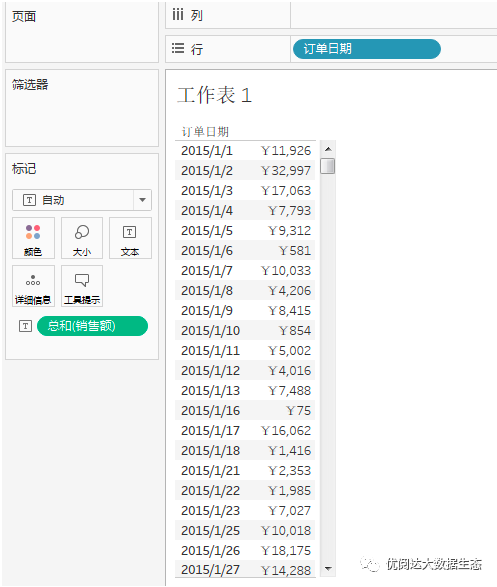There are a lot of date functions in Tableau. Today we share 7 functions for data powders to see how they help achieve dates.

The Tableau trick we want to share with you this issue is: learn several common date functions.

In order to facilitate learning, chestnut selection representative application scenarios, master the chestnut method, data powder can try further exploration.

Specific steps are as follows:

If you want to add a list of 3 months later, you can use the dateAdd function.

Create a calculation field, type a function: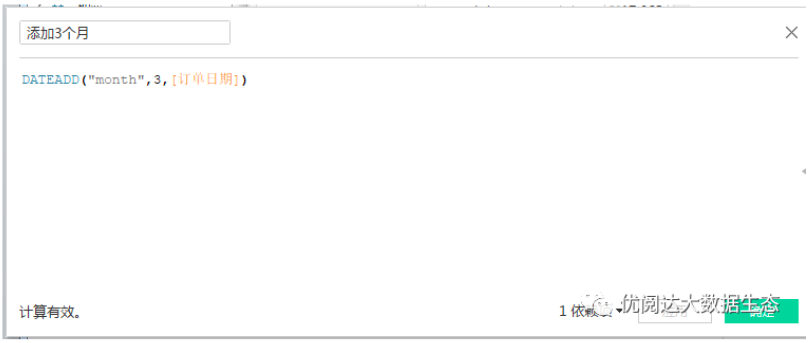At this time, the month of the date has been added a column, the date of 3 months later.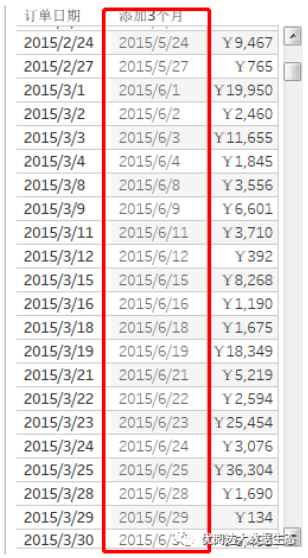No.2, dateDiff function

If you want to calculate the order from the place to the delivery, the calculation logic should be "shipping date" minus "order date". In this case, you can also use the Datediff function to return two dial differences, and obtain each order from the order to the delivery.

Create a calculation field, type a function:

Datediff ("day", [order date], [shipping date])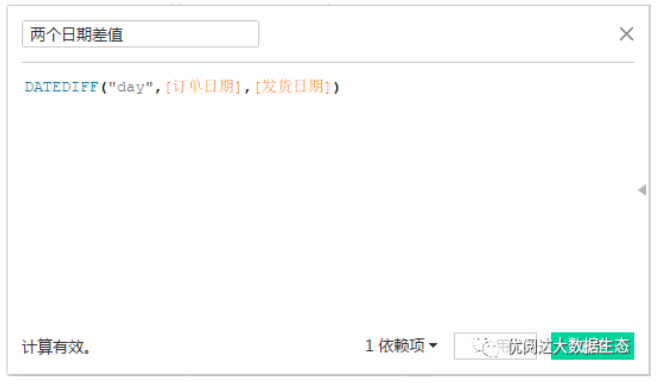In order to facilitate comparison, we will look along with the direct difference calculation results of the two dates.It can be seen that the value obtained by the two calculation methods is the same (the metric of the difference is changed to average).

Tips: Direct difference calculation of two dates, when Datediff's "DatePart" is established, because the two dates are directly subtracted, the result is only in the sky.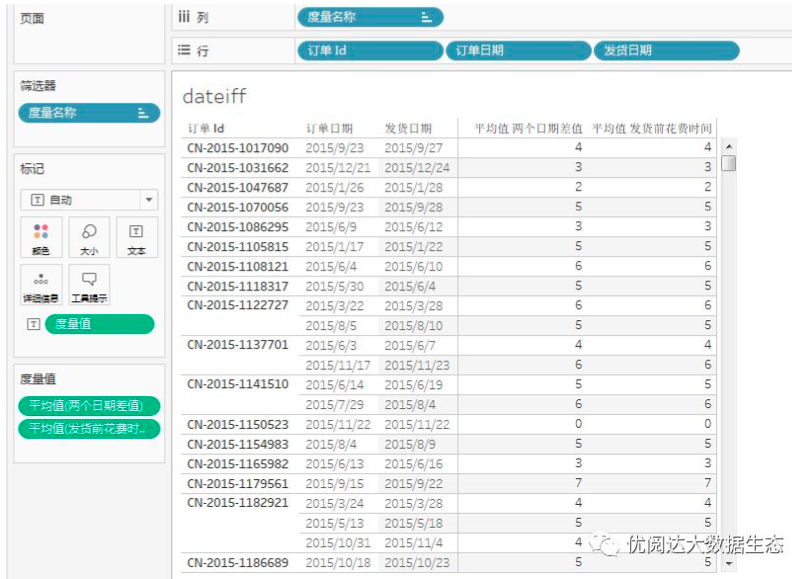No.3, DatePart function

If you want to get a single value (year, month, day), you can use the DATEPART function to calculate. If you want to get the month value in the order date,

Create a calculation field, type a function:

DatePart ("Month", [Order Date])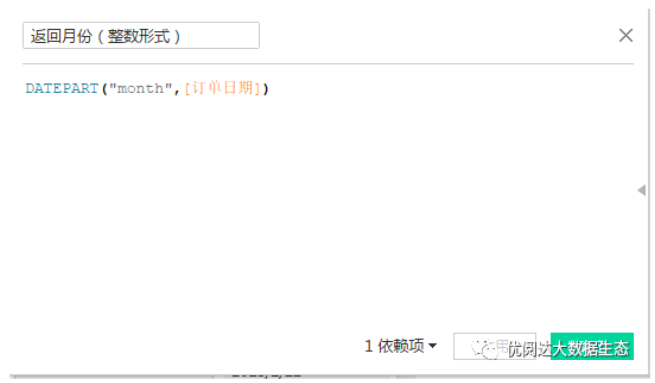It can be seen that the value of the order date in the chart, the value of each month, has been listed separately.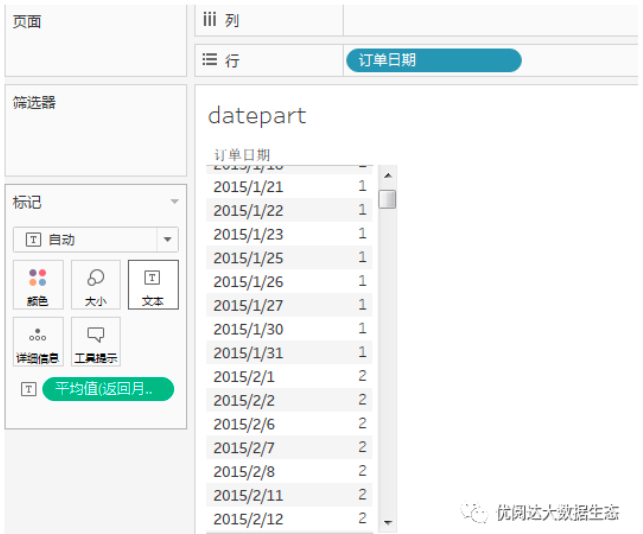No.4, datetrunc function

If you want to create a month or season on the date of the current date. You can use the Datetrunc function to create a new date value based on the existing date, such as the first day of each month.

Create a calculation field, type a function:

Datetrunc ("MONTH", [Order Date])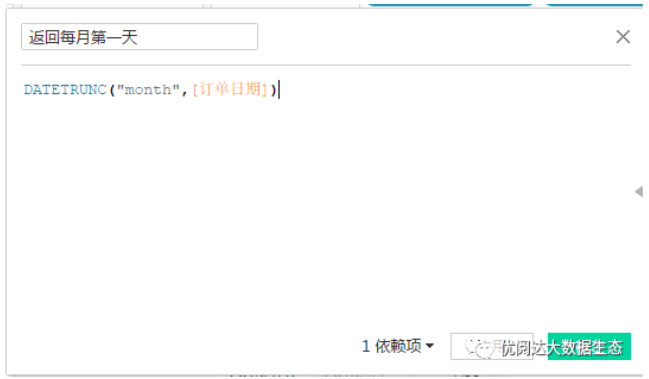On the right side of the order date in the chart, the first day of each month has been listed separately.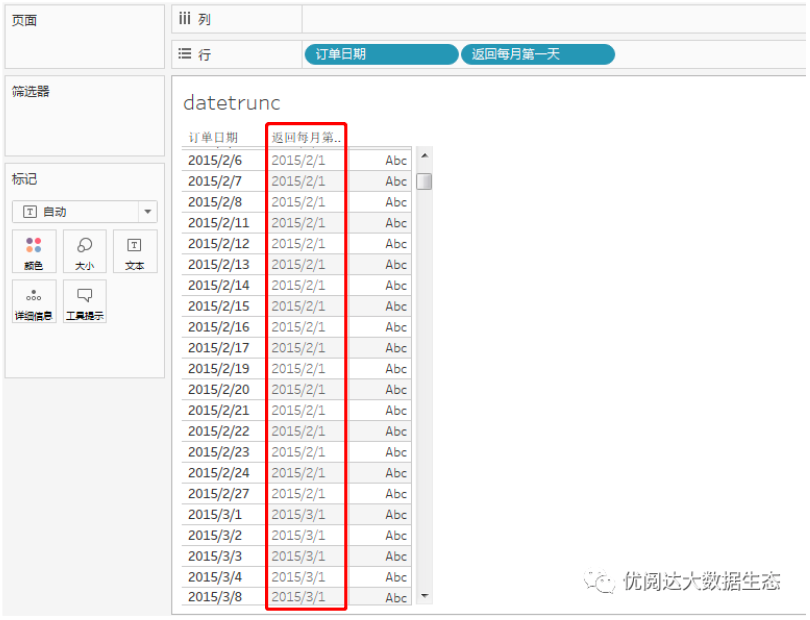No.5, Datename function

You can use the DateName function if you need to convert some part of the date to a string format. For example, convert the month into English list.

Create a calculation field, type a function:

Datename ("MONTH", [Order Date])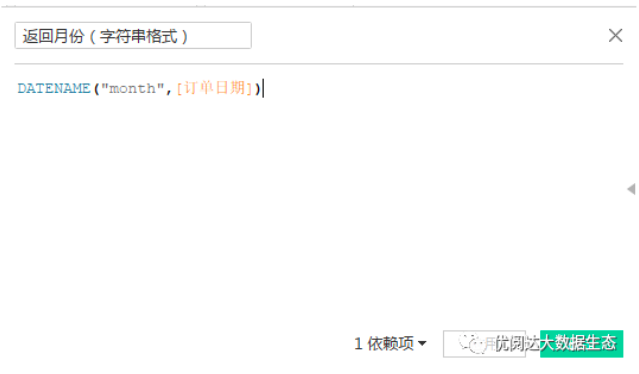The string format of the month will be returned, and the field of the English in English will appear in the chart.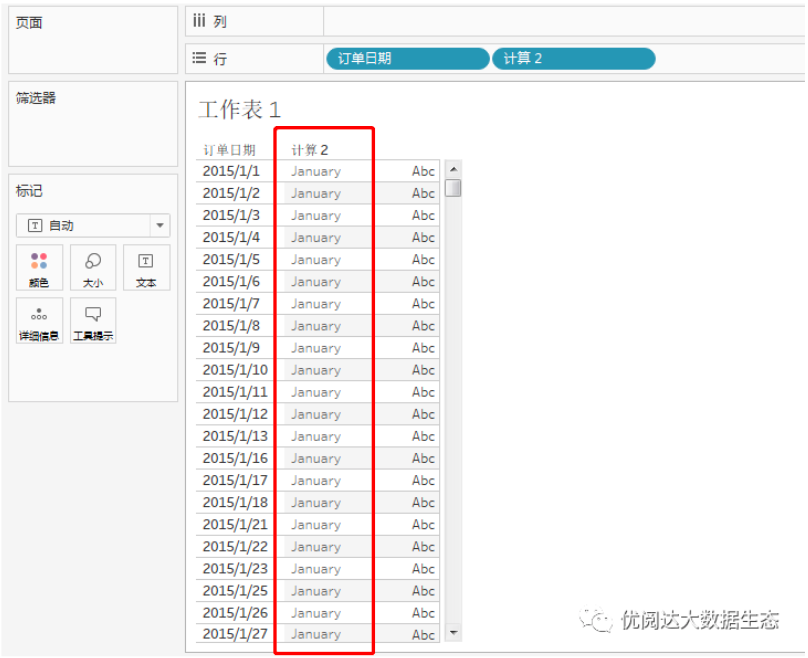No.6, Datepharse function

Of course, we can also convert existing strings to the date with the Datepharse function to the specified format.

First, we copy a order date and change to a string format.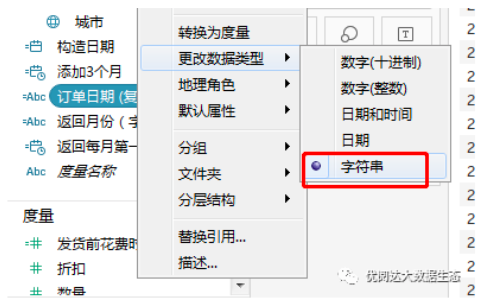Create a calculation field, type a function:

DateParse ("YYYY-MM-DD", [Order Date (Copy)])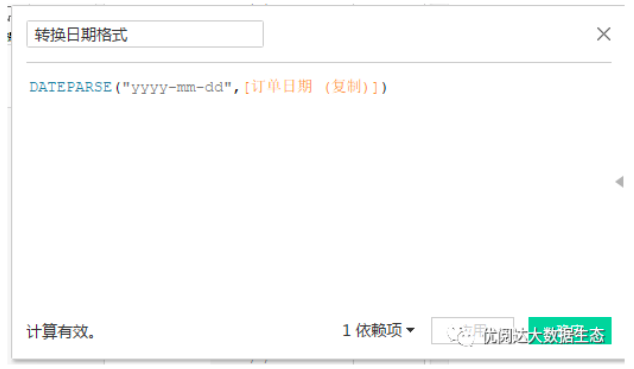The string is converted to a specific date format.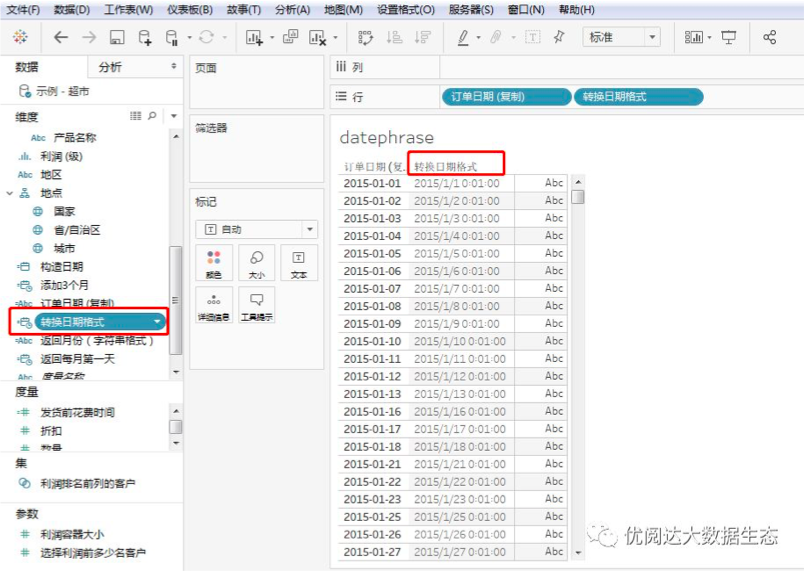No.7, Makedate function

If you want to return a date value based on the specified year, the month and date constructed, you can use the MakeDate function.

We use such a data source: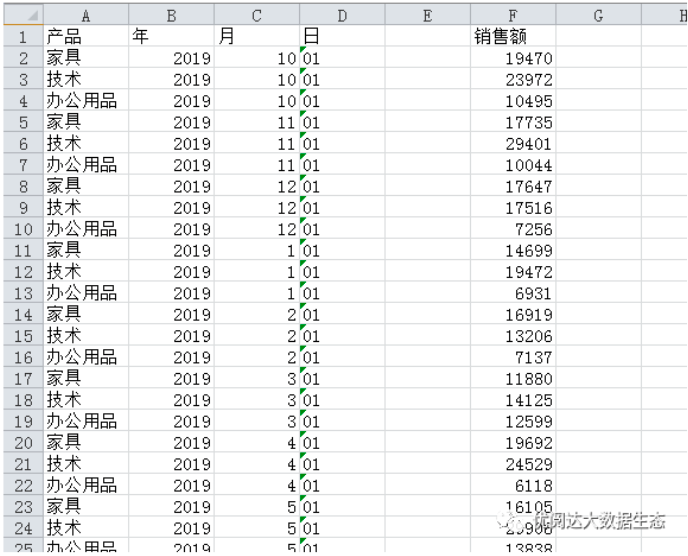They are present in the worksheet: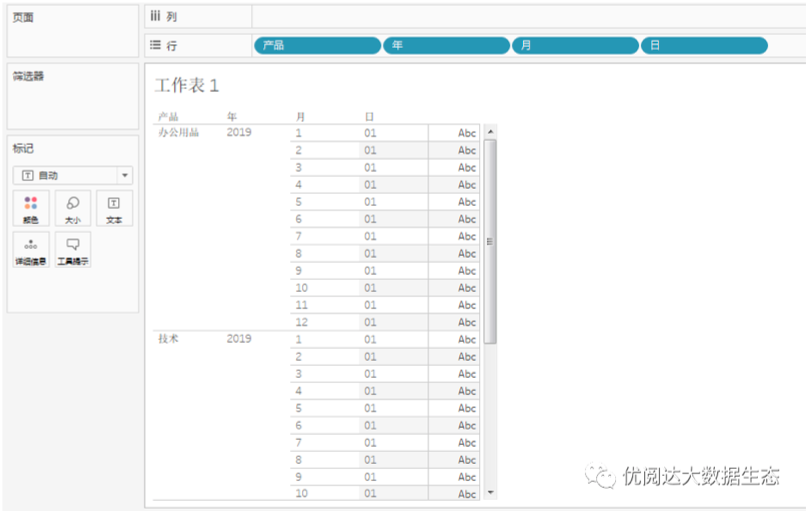We need a complete date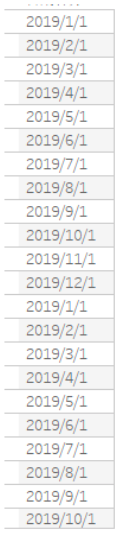This situation, it is recommended to use the MakeDate function, create a calculation field, type a function: makedate ([year], [month], [day])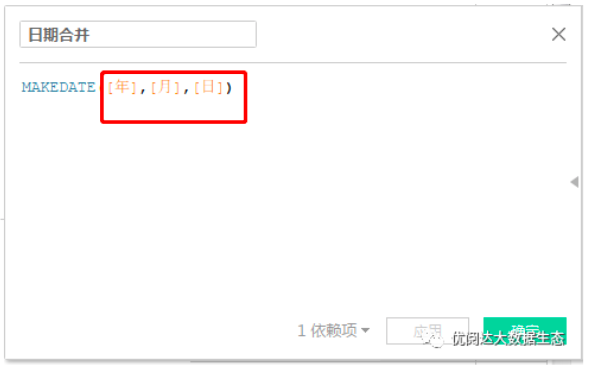Tips: The month is an integer format at this time.

In this way, the end of the completion date is completed.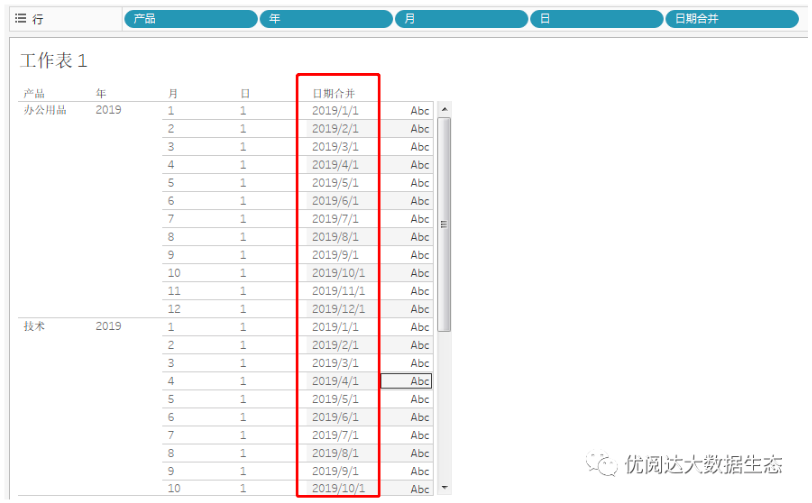This issue of Tableau skills, have you get? Try it quickly!